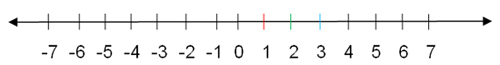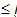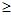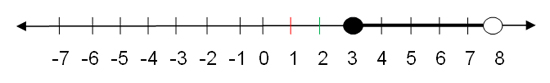How to Add, Subtract, Multiply, and Divide Positive and Negative Numbers

 Negative Numbers

Let's look at the following number line and notice that each point (dot) on the number line corresponds to one number:On the number line above, we can see three types of numbers, or integers: negative numbers, zero, and positive numbers. The negative numbers are to the left of zero, so they are less than zero. The positive numbers are to the right of zero, so they are greater than zero. Zero, the dividing point, is neither positive nor negative.

For the number line above, "1" corresponds or is related to the red point, "2" is related to the green point, "3" is related to the blue point, and so forth. When we move to the right on the number line, we increase in numbers. We defined this as addition. When we move to the left, we decrease. And we defined this as subtraction. This is generally the way a number line works.

When we add two positive numbers or multiply two positive numbers, we get a positive number. However, we can subtract a positive number from a positive number and suddenly, we don't get a positive number!

For example, if we subtract 7 from 4, we would start at 4 on the number line and move left 7 places. That brings us to -3. Because -3 is to the left of 0, it is less than zero.

Looking at the inverse operation, we can say that if 4 – 7 = -3, then -3 + 7 = 4. And that is correct. If we start at -3 and move 7 spaces right, we will be at 4.

Positive numbers are not just the integers to the right of the zero, but all types of numbers like fractions, decimals, and radicals. Negative numbers also include various forms and various types of numbers that appear left of the zero.

We don't always have a number line with which to work, so we need to learn a few rules about working with negative numbers. First, we need to define absolute value. The absolute value of a number is the number of units the number is from zero. It is always expressed as positive but without a ‘plus' sign.

The absolute value of 3 is 3. The absolute value of -3 is also 3. Both 3 and -3 are three units from zero. Absolute value is denoted by writing the number between two vertical bars.

|3| = 3 and |-3| = 3

If you don't see a negative or positive sign in front of a number, it is positive.

When adding numbers of the same sign (either positive or negative), add their absolute values and give the result the same sign.

6 + 5 = 11 (6 and 5 are both positive; 6 + 5 is 11 which is positive)

-7 + -8 = -15

(-7 and -8 are both negative; add |7| + |8| which is 7 + 8 to get 15; the answer is -15)

If all the numbers in a group to be added are negative: -2 + -3 + -4 = -9, again add the absolute values of 2 + 3 + 4 to get 9 and give it a negative sign.

When adding numbers of the opposite sign, take their absolute values, subtract the smaller from the larger, and give the result the sign of the number with the larger absolute value.

7 + -3 = |7| – |3| = 4

-8 + 6 = |8| which is 8 and |6| which is 6. Subtract the smaller from the larger:

8 – 6 which gives a result of 2 and give it the sign of the larger number which is 8.

 Subtracting Positive Numbers and Negative Numbers

When subtracting a positive number from a negative number, use the same rule as for adding two negative numbers: add the absolute values and give the difference a negative sign.

-5 - 4 = |5| + |4| = |9| = -9 (this is like -5 + -4 = -9)

-2 – 12 = |2| + |12| = |14| = -14

When subtracting a negative number from a positive number, the double negative of subtracting a negative becomes a positive, so use the same rule as for adding two positive numbers: add the absolute values and give the difference a positive sign.

Interested in learning more? Why not take an online Basic Math course?

5 – -4 = |5| + |4| = 5 + 4 = 9

If you were to use a number line, you would go left for subtract and then reverse (to the right) for the negative number, so the final answer is to the right of the original number.

16 – -10 = |16| + |10| = 16 + 10 = 26

A number's additive inverse is the number of the opposite sign such that when the two are added, the result is zero.

a + (-a) = 0

As you can see this is the positive and negative numbers of the same absolute value.

10 + -10 = 0

-24 + 24 = 0

 Multiplying Positive and Negative Numbers

When multiplying a positive number and a negative number (or a negative number and a positive number), multiply the absolute values, and give the answer a negative sign.

8 x -5 = |8| x |5| = 8 x 5 = 40, but give it the negative sign, making it -40

-13 x 3 = -39

9 x -3 = -27

To multiply multiple numbers, count the number of negative signs on the numbers to be multiplied. If it is an even number, the product will be positive, and if it is an odd number, the product will be negative.

6 x -2 x -3 x 5 = |6| x |2| x |3| x |5|

6 x 2 = 12, 12 x 3 = 36, and 36 x 5 = 180

There are two negative signs (an even number), so the answer is positive.

If it had been -6 x -2 x -3 x 5, the answer would have been -180

 Multiplying Two Negative Numbers

When multiplying two negative numbers, the two negatives cancel each other, so multiply the absolute values, and give the answer a positive sign.

-21 x -3 = |21| x |3| = 63 (which remains positive)

-7 x -8 = |7| x |8|   = 56

 Dividing a Negative Number by a Negative Number

To divide two numbers that have the same sign (either two positives or two negatives), use the absolute values and the result will be positive.

16 ¸ 4 = |16| ¸ |4| = 4

-20 ¸ -10 = |20| ¸ |10| = 2

Dividing a Positive Number by a Negative Number or a Negative Number by a Positive Number

To divide a pair of numbers that have different signs (negative by positive or positive by negative), use the absolute values of the two numbers, and give the result the negative sign.

-12 ¸  3 = |12| ¸ |3| = 4, but it is -4

18 ¸  -3 = |18| ¸  |3| = 6, but it is -6

 Uses of Negative Numbers

Negative numbers are used to show cold temperatures. Numbers below 0°C are negative and are below freezing. (Remember that numbers below 32°F are below freezing, but temperatures often go below 0°F.)

Negative numbers are used to show measurements below sea level. Sea level is given as 0.

Negative numbers are used with money to show indebtedness, or money owed. If a person or household spends more money than it makes, we say that they are "negative by a certain amount" or we call it "in the red" because accountants use red ink to show negative numbers.

 Greater Than and Less Than and Sets of Numbers

A set of numbers is a group of numbers that answers a given description. For example, the set of integers less than 0 would be expressed as n < 0. In this sentence, the set of numbers that satisfies the conditions would be all the negative integers.

All of the integers greater than 0 would be expressed as n > 0. The set of numbers that satisfies these conditions would be the set of all the positive integers. Each of these integers would be called a member or element of that set.

What are the integers between 3 and 8? That would be 4, 5, 6, and 7. Another way to express this would be the set of numbers that is greater than 3 but less than 8, which can be put into a mathematical sentence that looks like:

3 < n < 8

Read this: n such that n is greater than 3 and less than 8

Because 3 < n or 3 is less than n or n is greater than 3

And n < 8 or n is less than 8 or 8 is greater than n

n = 4, 5, 6, 7

We could have said 3n < 8 in which case, 3 would have been included in the answer, so n = 3, 4, 5, 6, 7. Thesign means ‘less than or equal to' and thesign means ‘greater than or equal to.'

Often these ‘greater than' and ‘less than' answers need to be expressed using a number line because it would be impossible to list all the numbers for the answer.We make an open circle on the number if it is a ‘greater than' (>) or ‘less than' (<) and we make a filled circle on the number if it is a ‘greater than or equal to' () or a ‘less than or equal to' (). We darken the line from the beginning circle to either an ending circle or the point on the line.